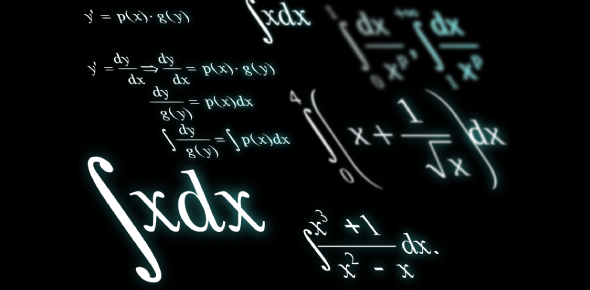# Differentiation Practice Questions With Answers

10 Questions | Attempts: 2553
ShareSettingsDo you really think you're good at solving every type of differentiation problem? Here, we have come up with differentiation questions with answers for you to make your understanding better. In mathematics, differentiation is a process and method of finding the derivative of a function. Here, you'll have to solve some of the hardest differentiation questions. Do you think you can do so? Try these differentiation practice questions and check your calculus skills. Do not forget to share the quiz.

• 1.
Which method should we use for y=(x-2)3(x+1)?
• A.

Chain rule

• B.

Product Rule

• C.

Quotient Rule

• D.

Substitution method

• 2.
Which method should use for y = x5/(x-3)?
• A.

Chain Rule

• B.

Product Rule

• C.

Quotient Rule

• D.

Gauss Method

• 3.
The differentiation of 7x-3 is 21x-4. True or False
• A.

True

• B.

False

• 4.
Differentiate y= -2x- 6x2 + 3.
• A.

12

• B.

2x4-12x2+3

• C.

10x4-12x

• D.

-10x4-12x

• 5.
Y=x4(x-2)4 can be solved by Quotient Rule. True or false
• A.

True

• B.

False

• 6.
Y= (x+4)/(x-3)4 can be solved by Quotient Rule. True or false
• A.

True

• B.

False

• 7.
Differentiate y= 6x - sin (3x).
• A.

3 cos (3x)

• B.

6 - 3 cos (3x)

• C.

6 + cox (3x)

• D.

6 - cos (3x)

• 8.
Differentiate y = 4x3 - e2x.
• A.

12x3- ex

• B.

12x2 - 2ex

• C.

12x- 2e2x

• D.

4x2 - 2e2x

• 9.
Differentiate y = x(x2-6).
• A.

6x

• B.

3x- 6

• C.

X2

• D.

X3 - 6x

## Related TopicsBack to top
×

Wait!
Here's an interesting quiz for you.